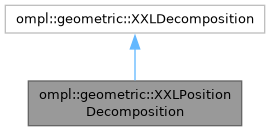ompl::geometric::XXLPositionDecomposition Class Referenceabstract
Inheritance diagram for ompl::geometric::XXLPositionDecomposition:[legend]

## Public Member Functions

XXLPositionDecomposition (const base::RealVectorBounds &bounds, const std::vector< int > &slices, bool diagonalEdges=false)

virtual int getNumRegions () const
Returns the number of regions in this XXLDecomposition.

virtual int getDimension () const
Return the dimension of this HiLoDecomposition.

virtual int numLayers () const =0
Return the number of layers possible in this decomposition. Must be at least 1.

virtual int locateRegion (const base::State *s) const
Return the id of the region that this state falls in.

virtual int locateRegion (const std::vector< double > &coord) const
Return the id of the region that this coordinate falls in.

virtual void getNeighbors (int rid, std::vector< int > &neighbors) const
Stores the given region's neighbors into a given vector.

virtual void getNeighborhood (int rid, std::vector< int > &neighborhood) const
Stores the given region's neighbors into the vector. This returns the 8-connected grid neighbors of the cell, regardless of whether diagonal edges exist.

virtual double distanceHeuristic (int r1, int r2) const
An admissible and consistent distance heuristic between two regions. Manhattan distance on grid.

virtual bool sampleFromRegion (int r, base::State *s, const base::State *seed=nullptr) const =0
Sample a state s from region r in layer 0.

virtual bool sampleFromRegion (int r, base::State *s, const base::State *seed, int layer) const =0
Sample a state s from region r in the given layer.

virtual void project (const base::State *s, std::vector< double > &coord, int layer=0) const =0
Project the given State into the XXLDecomposition.

virtual void project (const base::State *s, std::vector< int > &layers) const =0
Project the state into the decomposition and retrieve the region for all valid layers.

void ridToGridCell (int rid, std::vector< int > &cell) const

int gridCellToRid (const std::vector< int > &cell) const
Return the region id corresponding to the (discrete) grid cell coordinates.

int coordToRegion (const std::vector< double > &coord) const
Return the region id of the given coordinate in the decomposition.

int coordToRegion (const double *coord) const

bool hasDiagonalEdges () const
Return true if the decomposition has diagonal edges.Public Member Functions inherited from ompl::geometric::XXLDecomposition
XXLDecomposition ()
Constructor.

virtual bool canSteer () const
Returns true if the method steerToRegion is available.

virtual bool steerToRegion (int, int, const ompl::base::State *, std::vector< ompl::base::State * > &) const

## Protected Member Functions

void getNonDiagonalNeighbors (int rid, std::vector< int > &neighbors) const

void getDiagonalNeighbors (int rid, std::vector< int > &neighbors) const

## Protected Attributes

base::RealVectorBounds bounds_

const std::vector< int > slices_

std::vector< double > cellSizes_

bool diagonalEdges_

int numRegions_

## Detailed Description

Definition at line 113 of file XXLPositionDecomposition.h.

The documentation for this class was generated from the following files: